# $+\infty=-\infty$

Recently, a comment made by Matt Parker on a Numberphile video made me start to think about the implications of positive infinity equaling negative infinity.

First off, what would the number line look like? If $+\infty=-\infty$, then we could represent the number line as a number "circle", with both ends wrapping around to meet at the single point of infinity, like this: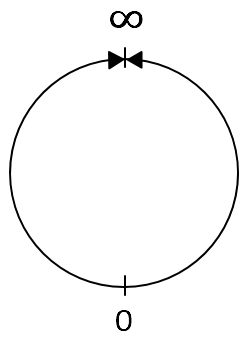This already feels very intuitive, because an infinite distance from $0$, whether you go in one direction or the other, seems as if it should be treated as just one distance: infinity.

But things get even more interesting!

How would a coordinate plane look if $+\infty=-\infty$? I propose that it would look like a torus: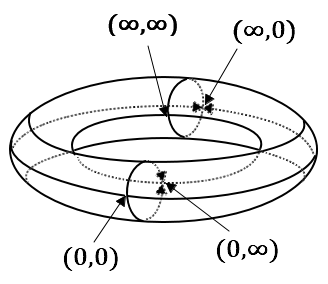At first this may seem confusing, but there is a reason I chose the torus over what might seem more natural: a sphere.

On a sphere, both the $x$ and $y$ axes would intersect at a single point of infinity. However, there are $3$ combinations of $0$ and $\infty$ which must be accounted for: $(0, 0)$, $(0, \infty)$, and $(\infty, \infty)$. A sphere would not be able to distinguish between these $3$ points, whereas a torus could. These points are all labelled in the picture above.

One of the exciting results of this model is that division by zero now becomes defined.

A common example of division by zero being undefined is the graph of the function $f(x) = \frac{1}{x}$. The graph is shown below: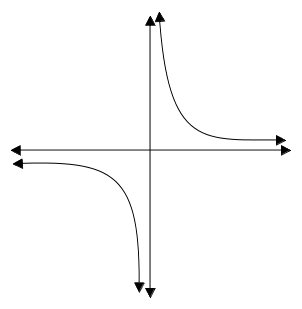As this function's input gets closer to $0$ from the right (the function getting closer to $\frac{1}{0}$), its output approaches $+\infty$, but as its input gets closer to $0$ from the left, its output approaches $-\infty$. Thus, on a coordinate plane, division by zero is undefined, since this graph doesn't approach one value for $\frac{1}{0}$.

But on a coordinate torus, this would not be an issue. Excluding the torus shape for clarity, the graph of the function $f(x) = \frac{1}{0}$ would look like this: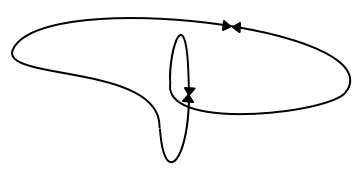Not only does this graph define division by zero since it approaches infinity from both directions, but it just looks beautiful! $:)$

I should note that the reason infinity is not commonly treated in this way is because it violates the axioms which make number sets such as the real numbers what is called a field. This means that infinity breaks the normal rules of arithmetic such as addition and multiplication so that proper algebra cannot be done with it.

However, I would propose that we adopt a single infinity into our fields and treat it in a special way. For instance, define $\frac{1}{0}$ or even $\frac{x}{0}$ (where $x \neq 0$) as equal to $\infty$, but define $\frac{0}{0}$ as undefined. This way, expressions such as $\infty \times 0$ remain undefined (since we can't derive them from $\frac{1}{0} = \infty$ without using an undefined expression first).

I should also note that the concept of a number "circle" and a coordinate "torus" only exist in infinity. On a finite level, all we can see is a number line and a coordinate plane. Notwithstanding this, it is still fun and practical to contemplate properties which only exist in infinity.

Does anyone else have any thoughts on the subject? I would love to hear them!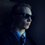Note by David Stiff
1 year, 6 months ago

This discussion board is a place to discuss our Daily Challenges and the math and science related to those challenges. Explanations are more than just a solution — they should explain the steps and thinking strategies that you used to obtain the solution. Comments should further the discussion of math and science.

When posting on Brilliant:

• Use the emojis to react to an explanation, whether you're congratulating a job well done , or just really confused .
• Ask specific questions about the challenge or the steps in somebody's explanation. Well-posed questions can add a lot to the discussion, but posting "I don't understand!" doesn't help anyone.
• Try to contribute something new to the discussion, whether it is an extension, generalization or other idea related to the challenge.
• Stay on topic — we're all here to learn more about math and science, not to hear about your favorite get-rich-quick scheme or current world events.

MarkdownAppears as
*italics* or _italics_ italics
**bold** or __bold__ bold
- bulleted- list
• bulleted
• list
1. numbered2. list
1. numbered
2. list
Note: you must add a full line of space before and after lists for them to show up correctly
paragraph 1paragraph 2

paragraph 1

paragraph 2

[example link](https://brilliant.org)example link
> This is a quote
This is a quote
    # I indented these lines
# 4 spaces, and now they show
# up as a code block.

print "hello world"
# I indented these lines
# 4 spaces, and now they show
# up as a code block.

print "hello world"
MathAppears as
Remember to wrap math in $$ ... $$ or $ ... $ to ensure proper formatting.
2 \times 3 $2 \times 3$
2^{34} $2^{34}$
a_{i-1} $a_{i-1}$
\frac{2}{3} $\frac{2}{3}$
\sqrt{2} $\sqrt{2}$
\sum_{i=1}^3 $\sum_{i=1}^3$
\sin \theta $\sin \theta$
\boxed{123} $\boxed{123}$

## Comments

Sort by:

Top Newest

Well, thats very interesting! Maybe you could build a field of numbers which includes infinity. I don't know how to do this, but I think this should be possible. I mean, the imaginary unit is just a special "number" which was pressed in the real numbers to get the complex ones ( to say it in the most unformal way ;) ). Even If you need to redefine the term field, I think it is possible. It took over 100 years to accept the complex numbers - So why shouldn't our childs accept this idea one day.

- 1 year, 6 months ago

Log in to reply

Thanks for the encouragement! :)

Unfortunately, based on my very limited knowledge of algebraic abstractions, I believe the way mathematicians have defined a "field" makes adding infinity to one impossible. But maybe there's a different type of abstraction which would work...

Great, now I'm going to have to research this! :)

- 1 year, 6 months ago

Log in to reply

As far as I am aware the (surreal numbers)[https://en.wikipedia.org/wiki/Surreal_number] form a field (besides of not being a set) and contain infinity-like numbers. Not in the sense of being the largest number, but they are larger than any real number. Of course these numbers don’t share properties such as $2\cdot \infty = \infty$ or $\frac{1}{\infty}=0$.

- 1 year, 3 months ago

Log in to reply

Thanks for the link Simon. I must admit, I am not well-versed in concepts such as set theory, but I have certainly heard of infinitesimals. In my uninformed opinion, infinitesimals seem to be just equal to $0$, but perhaps a different interpretation could be useful. Also just a quick editing remark: I believe you meant to put square brackets around the text, and parentheses around the link. :)

- 1 year, 3 months ago

Log in to reply

I have thought of this too, but it seemed very philosophical, not something I would write anywhere in mathematics. Reminds me of the saying: "As high as low" in the book of the "Lost Symbol" (bestseller). This book treats a kind of philosophy which partially is relative to this of what you just wrote (I recommend to read it if you like philosophy yourself). Even if it is fitting with our reality, it is not something we have time to study it (unless a crazy Astrophysicist is trying to use complex math for this... hypothesis).

Maybe infinity * ( -1 ) == infinity, maybe that undeterminable forms (0/0, inf / inf, etcetera...) have a solution, probably more than one... but that is something we leave to the future.

To me "As high as low" is like this: Imagine the most Egoist man in the world, so egoist that he understands that if I were to treat people nicely, in the future they would give me something back when I cannot have it, "I use them like this!".

Tell me your opinion.

- 1 year, 4 months ago

Log in to reply

That's interesting Nickolas. Though I'm not very interested in philosophy, the "as high as low" concept seems to describe this. I like to think of $\infty$ as simply meaning "unattainable". Thus, a positive or negative sign merely means which way we go to get there.

- 1 year, 3 months ago

Log in to reply

This page is soooooooooo useful in learning actually,it has a lot of explanation and the point is really interesting!Awesome

- 1 year, 3 months ago

Log in to reply

cool

- 1 year, 3 months ago

Log in to reply

Glad you enjoyed it! It's really more speculation than rigorous proof, but I thought it might be something worth sharing.

- 1 year, 3 months ago

Log in to reply

This is actually a really common way (well, not the torus) to consider the complex numbers. Rather than having a circle of infinities corresponding to each angle, there is just a single infinity. Then you can visualize the complex plane as a sphere. A point on the complex plane maps to a point on the unit sphere (which we center at (0,0,0)) by finding the intersection of [a line from the top of unit sphere to the point on the plane] with the sphere. https://en.wikipedia.org/wiki/Riemann_sphere

- 1 year, 3 months ago

Log in to reply

Thanks for the feedback Alex! I think I realize know why I came up with a torus instead of a sphere. On a 2 dimensional coordinate plane, we need the 2 coordinates which describe every point to remain distinct. Thus, we have to have a point for each combination of $0$ and $\infty$. But in the complex plane, each point can be represented as a single complex number and thus we only need one $\infty$. I will definitely check out that wikipedia article. It seems very pertinent to this discussion!

- 1 year, 3 months ago

Log in to reply

I think this makes a lot of sense! It's something I've thought about before too, and something in particular I like about it is the way it seems to complete the symmetry between 0 and infinity. I like to think of them as being counterparts to each other, since a lot of their properties are actually strikingly similar to each other (they just work in opposite ways). But where this symmetry traditionally fails is that we don't tend to think of negative 0 and positive 0 as being distinct from each other. Rather, this notion only really comes into play whenver we want to indicate a direction from which 0 is approached. I think infinity can work in a similar way :)

- 1 year, 2 months ago

Log in to reply

Thanks so much Daniel! That is exactly the way I think of it as well: signs merely indicate direction. Everyone uses directions when on their way towards a destination, but when they arrive, it makes no sense! $0$ and $\infty$ in this case are the destinations, for one cannot have more than everything and less than nothing. :)

This scenario is for graphing on two axes, but as it turns out, a related solution has long been known when using the complex plane, called the Riemann sphere. Thanks to @Alex Li for mentioning this. He provided a link in his comment, but here it is again.

- 1 year, 2 months ago

Log in to reply

Cool!

- 1 year, 1 month ago

Log in to reply

For all those interested, I've recently learned of a well documented algebra known as Wheel theory which satisfactorily incorporates the concept of division by zero for all numbers, including zero itself. It makes reference to a number "circle", usually referred to as the projectively extended real line, though not what I would consider to be it's 3-dimensional analog, the x-y torus. :)

- 2 weeks, 1 day ago

Log in to reply

It have been an axiom in algebra (and calculus, coordinate geometry, etc.) that the x-y plane is a $2$ dimensional entity, so if we defy it (as you have done assuming it as a torus) then you are breaking the fundamentals (of mathematics) which leads to a contradiction!

- 9 months, 2 weeks ago

Log in to reply

Even if it hurts given axioms and results in a contradiction, it does not mean that the idea is bad. There are plenty of new ideas in the history of mathmatics, where lots of mathmaticians believed it is impossible and will lead to a contradiction (e.g. invention of infinitesimals; invention of complex numbers). The idea just has to be very well structured, otherwise it will fail.

But now something else: What if we don't assume that the xy-plane is a torus, but we take an infinite big one and just map/project the xy-plane onto it?

- 9 months, 2 weeks ago

Log in to reply

No new idea gave rise to a contradiction, no axiom says that complex number aren't possible, and the idea of limits have taken of the idea of infinitesimal now it's of no use. Also it have been an axiom that even if you go infinitely far in the x-y plane, even then it is a plane which is straight not torus. It's name itself says x-y plane.

- 9 months, 2 weeks ago

Log in to reply

@CodeCrafter 1 @Zakir Husain Though not directly inspired by it, I realized later on that the "number circle" I had postulated actually had a name: the projectively extended real line. I guess you could say the x-y "torus" is an extension of this concept. :)

- 9 months, 2 weeks ago

Log in to reply

Then let's maybe call this weird number system where infinity equals minus infinity "the x-y torus".

- 2 weeks, 1 day ago

Log in to reply

×

Problem Loading...

Note Loading...

Set Loading...